# NCERT Solutions for Class 9 Science Chapter 4 – Structure of the Atom

NCERT Solutions for Class 9 Science Chapter 4 – Structure of the Atom, contains solutions to various questions in Exercises for Chapter 4. Structure of the Atom Class 9 NCERT Solutions have been explained in a simple and easy to understand manner. We are providing all subjects which can be accessed by clicking here.

## Download NCERT Solutions for Class 9 Science Chapter 4 – Structure of the Atom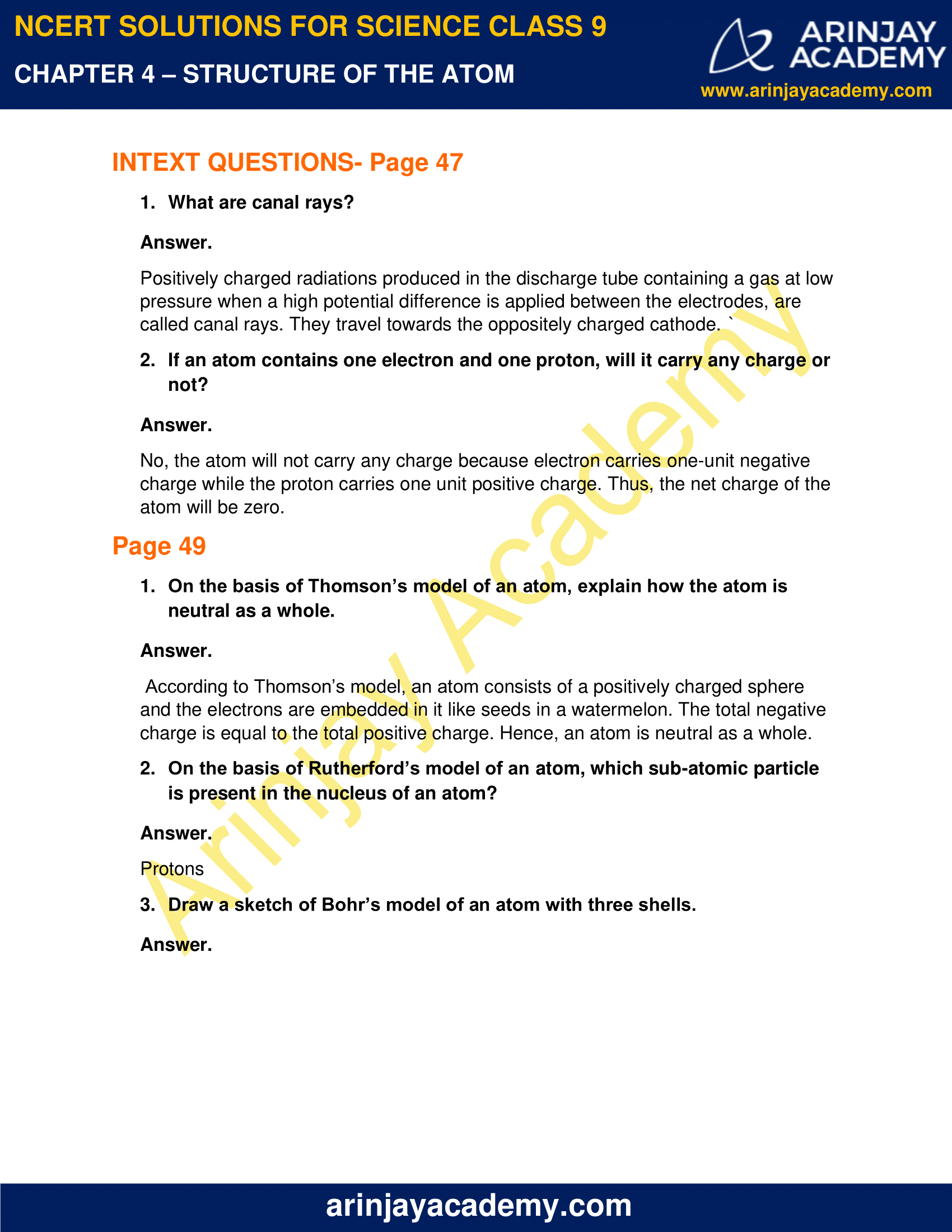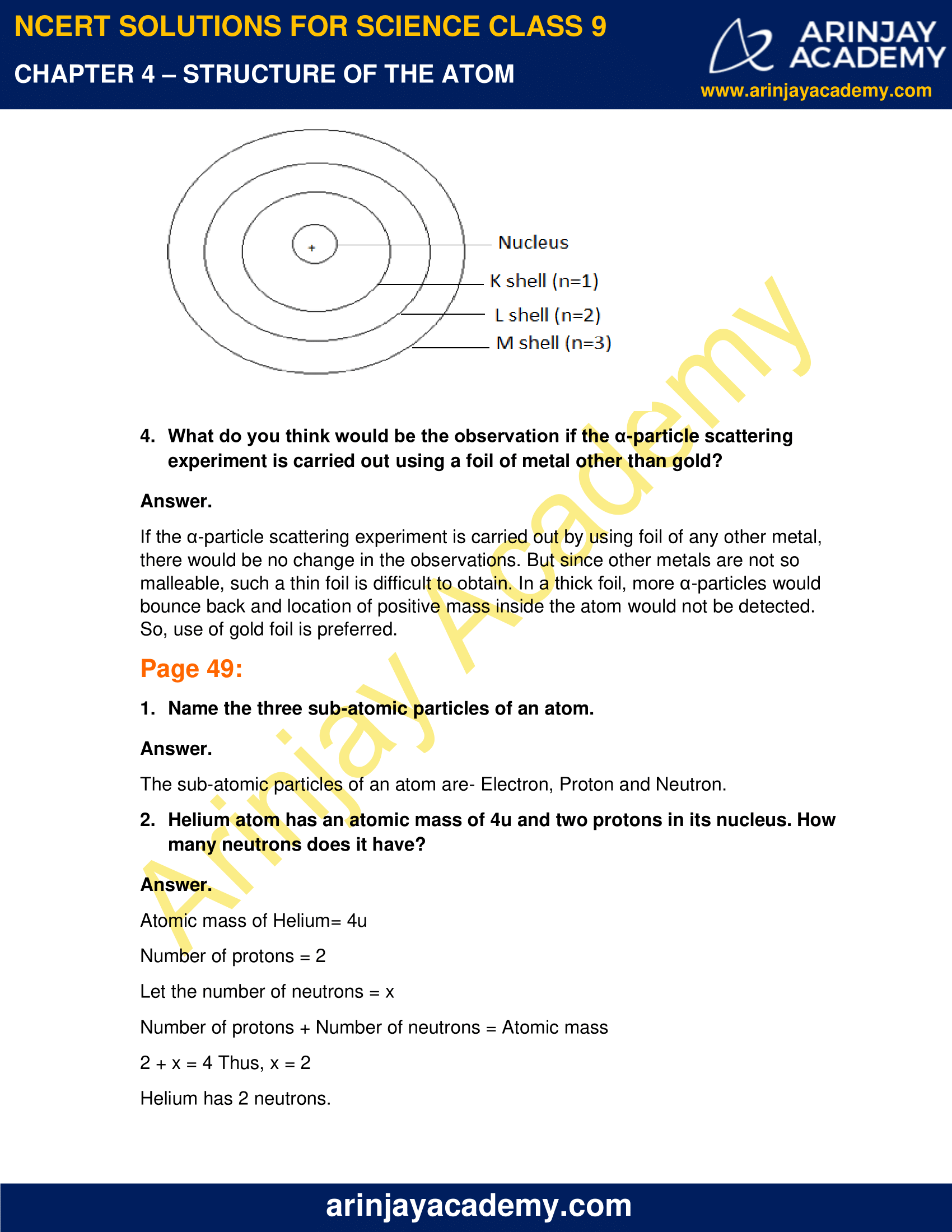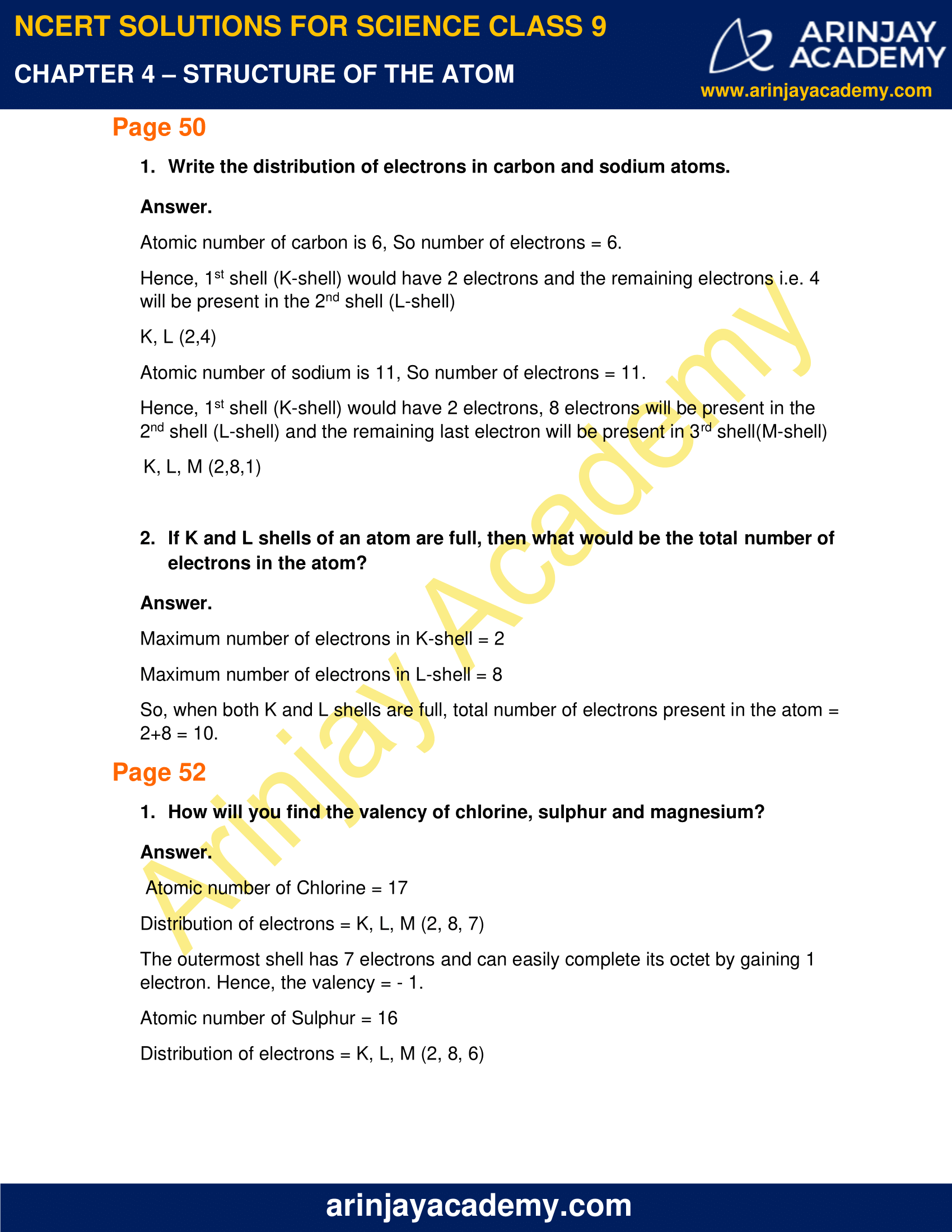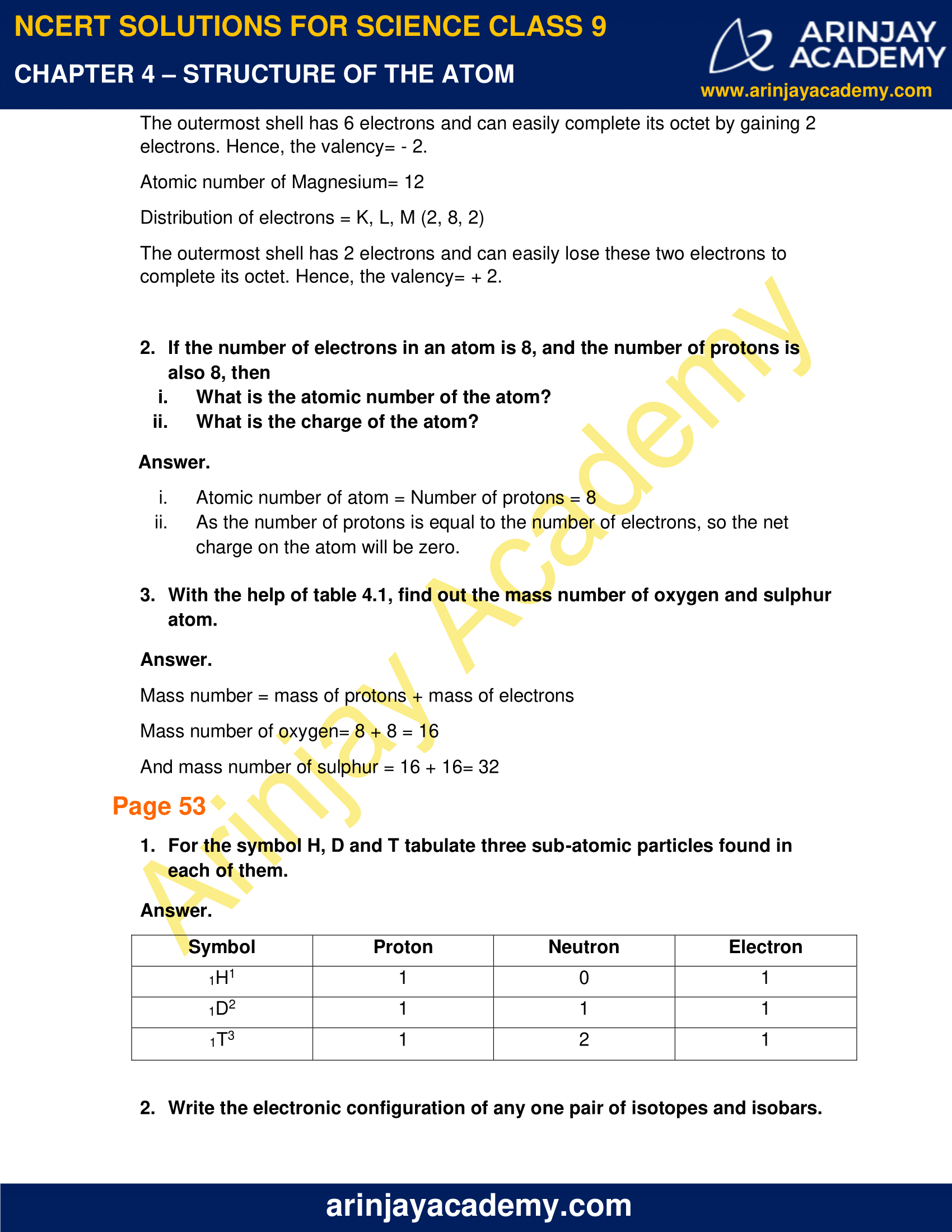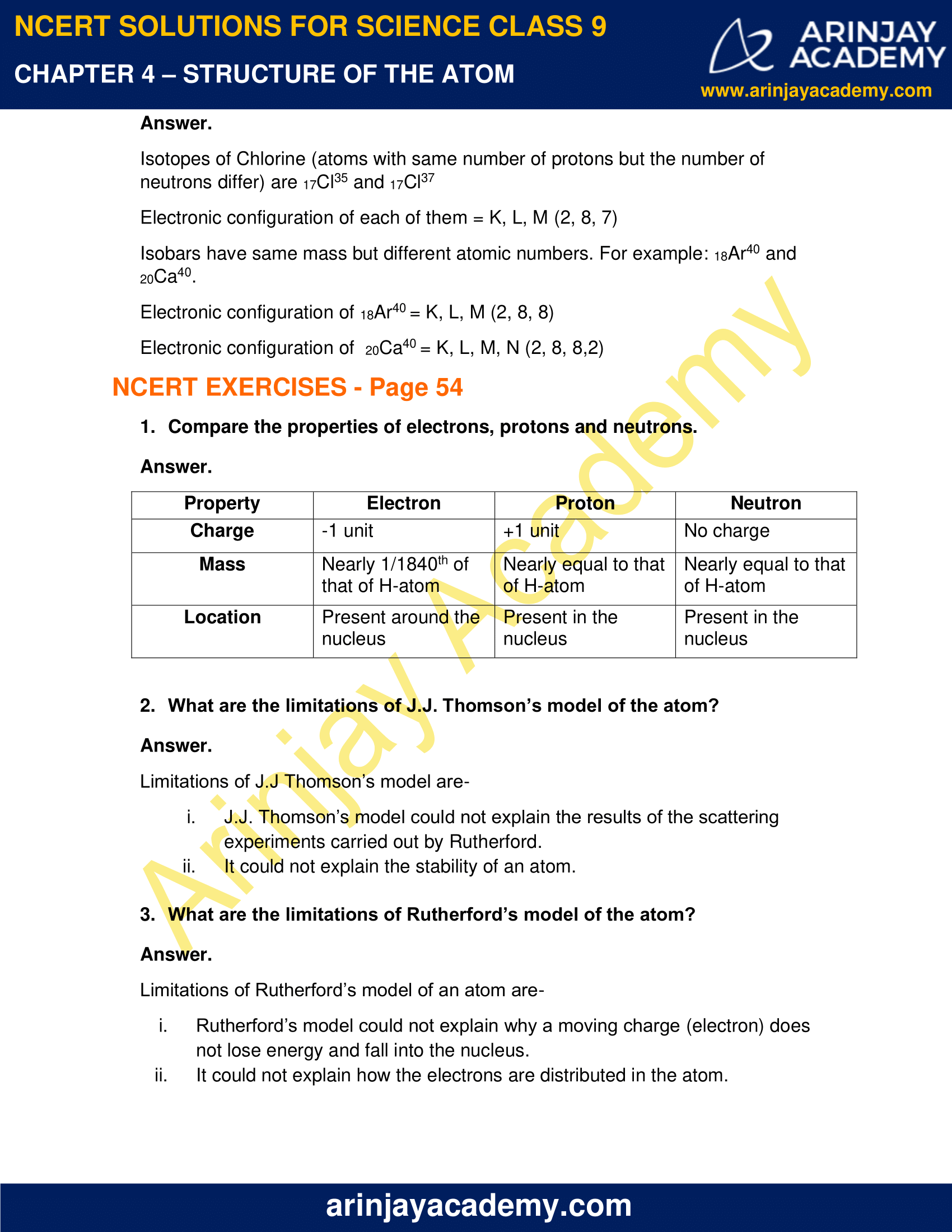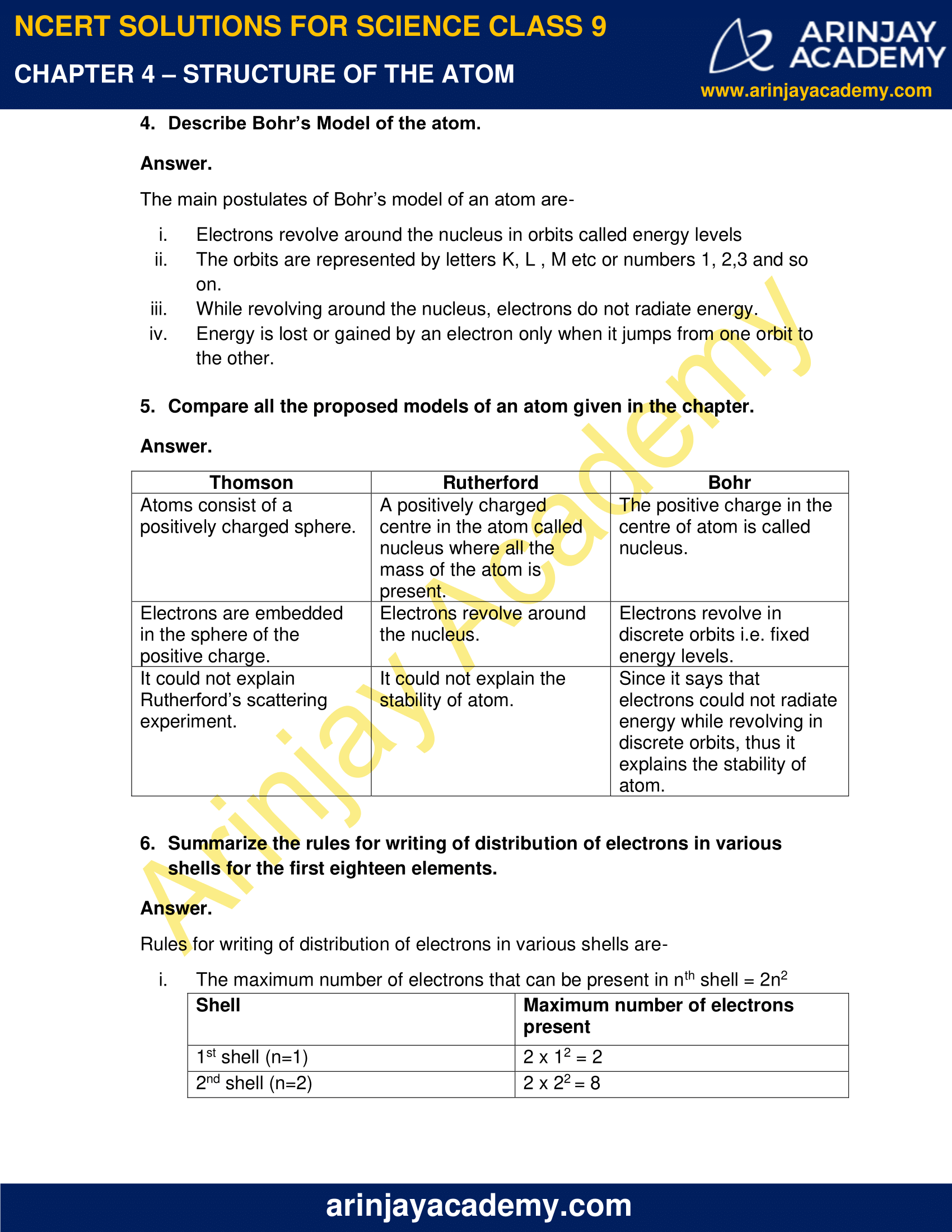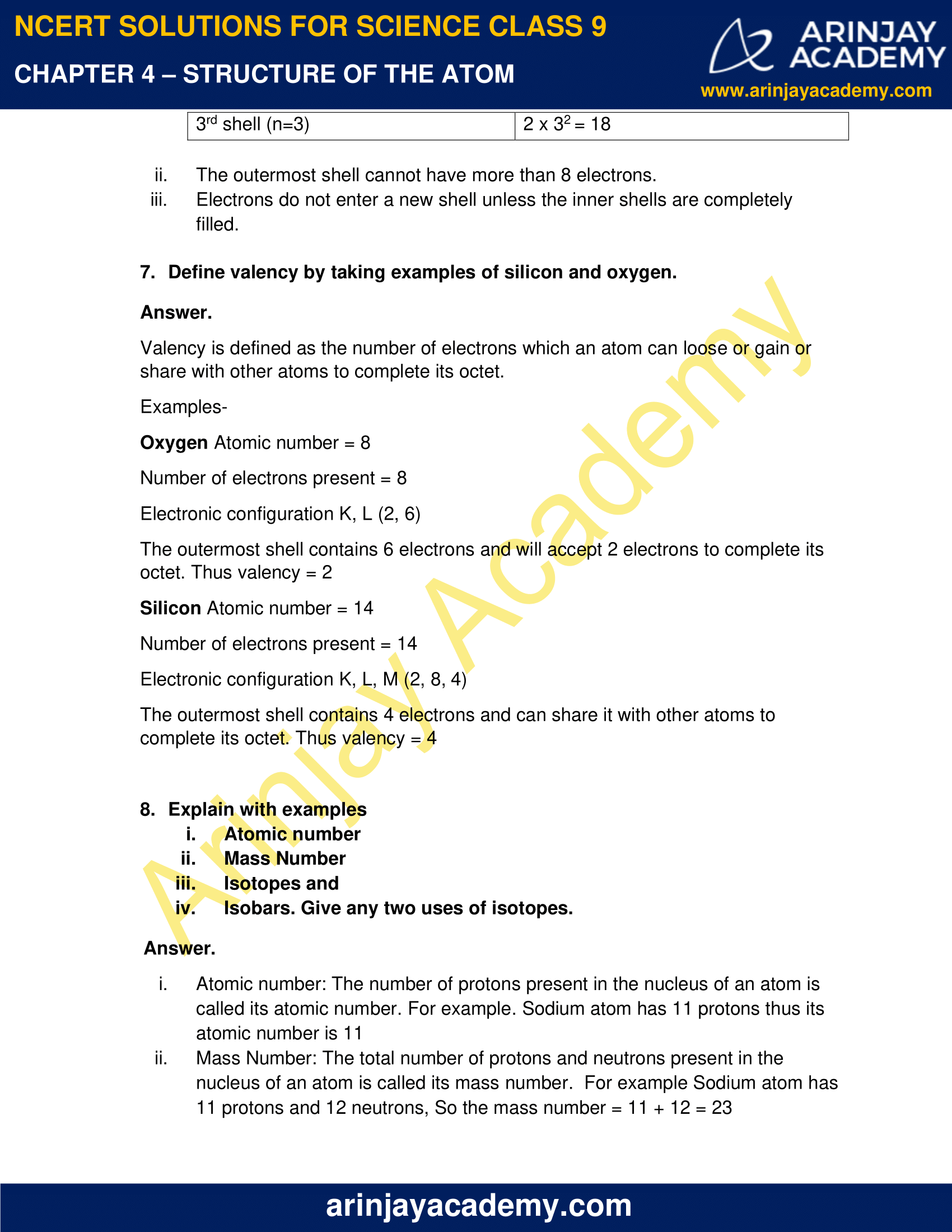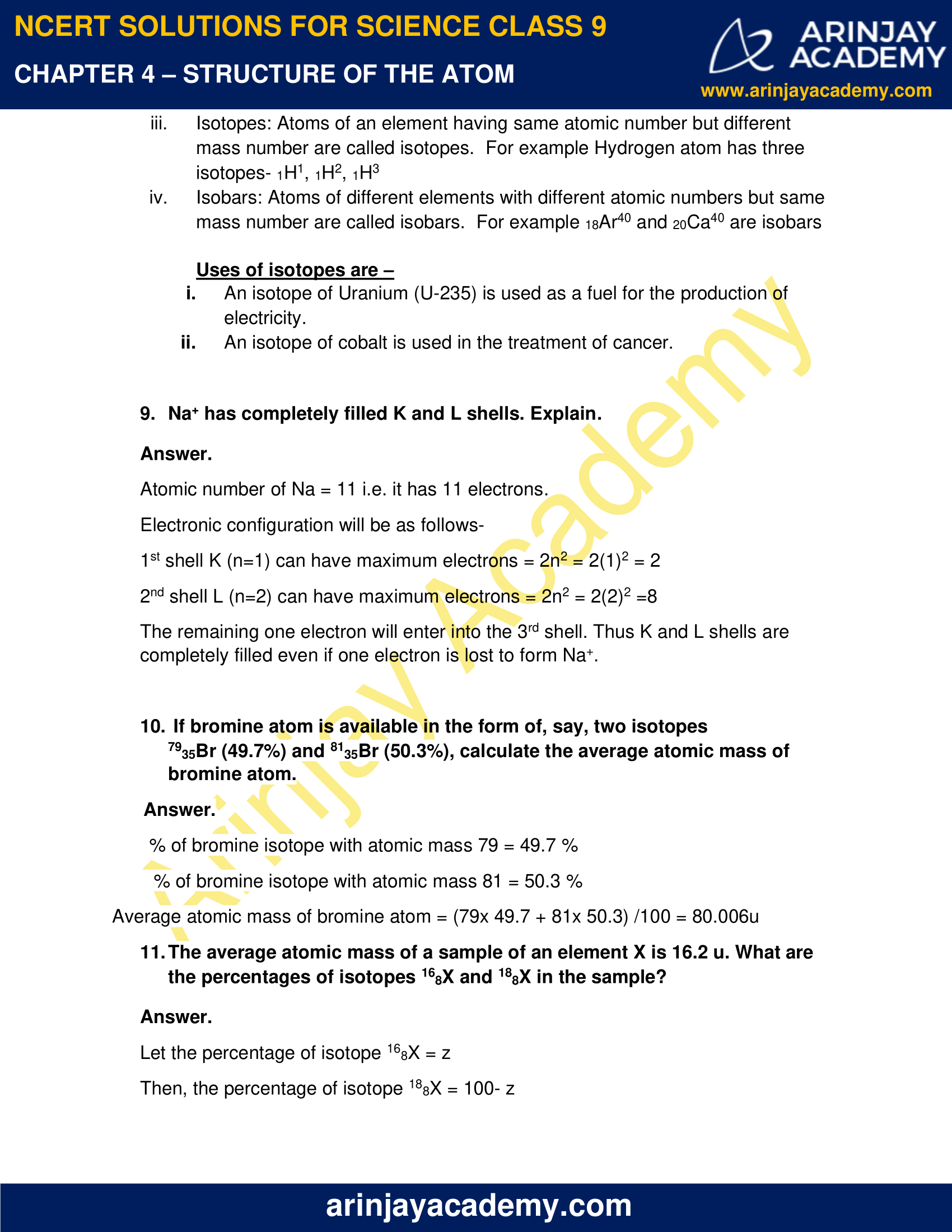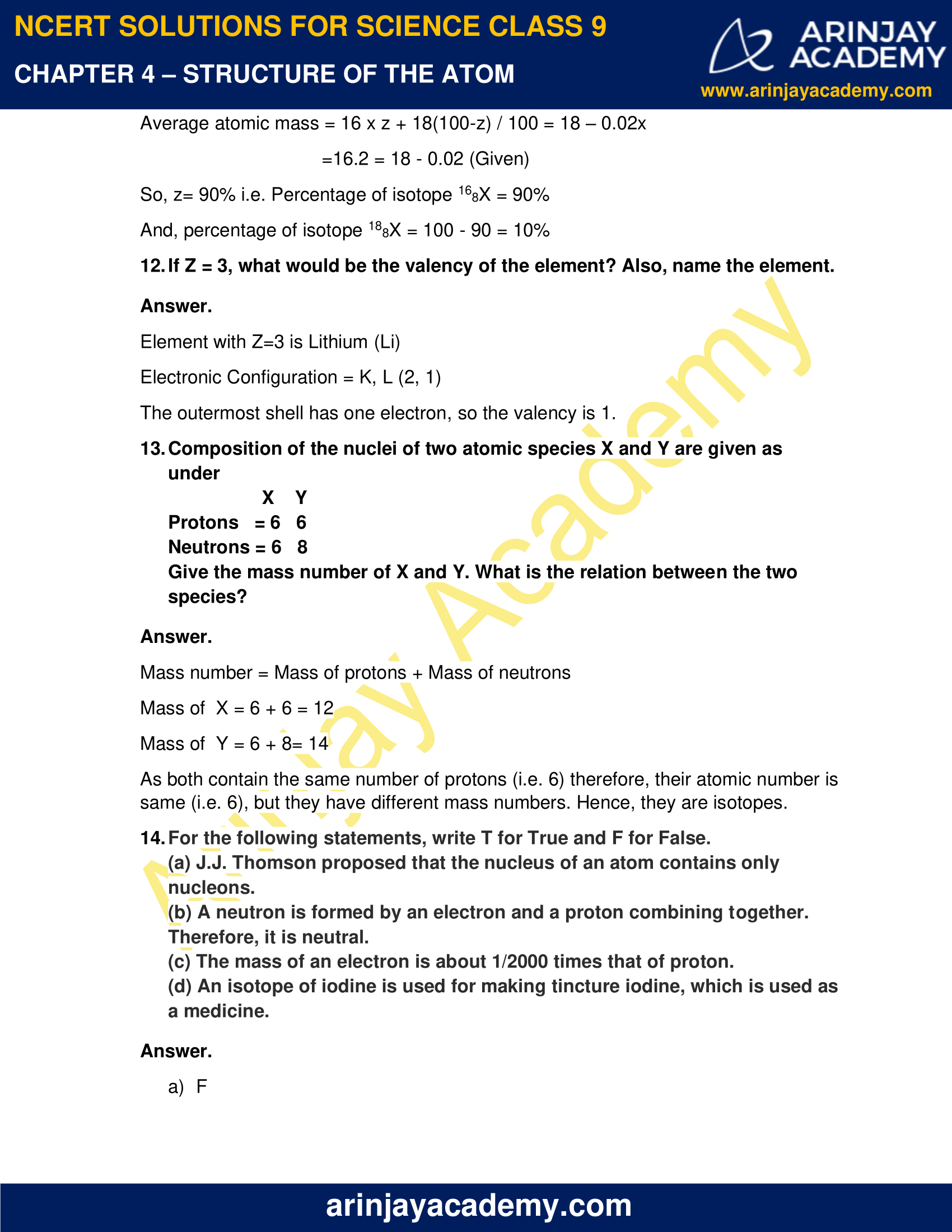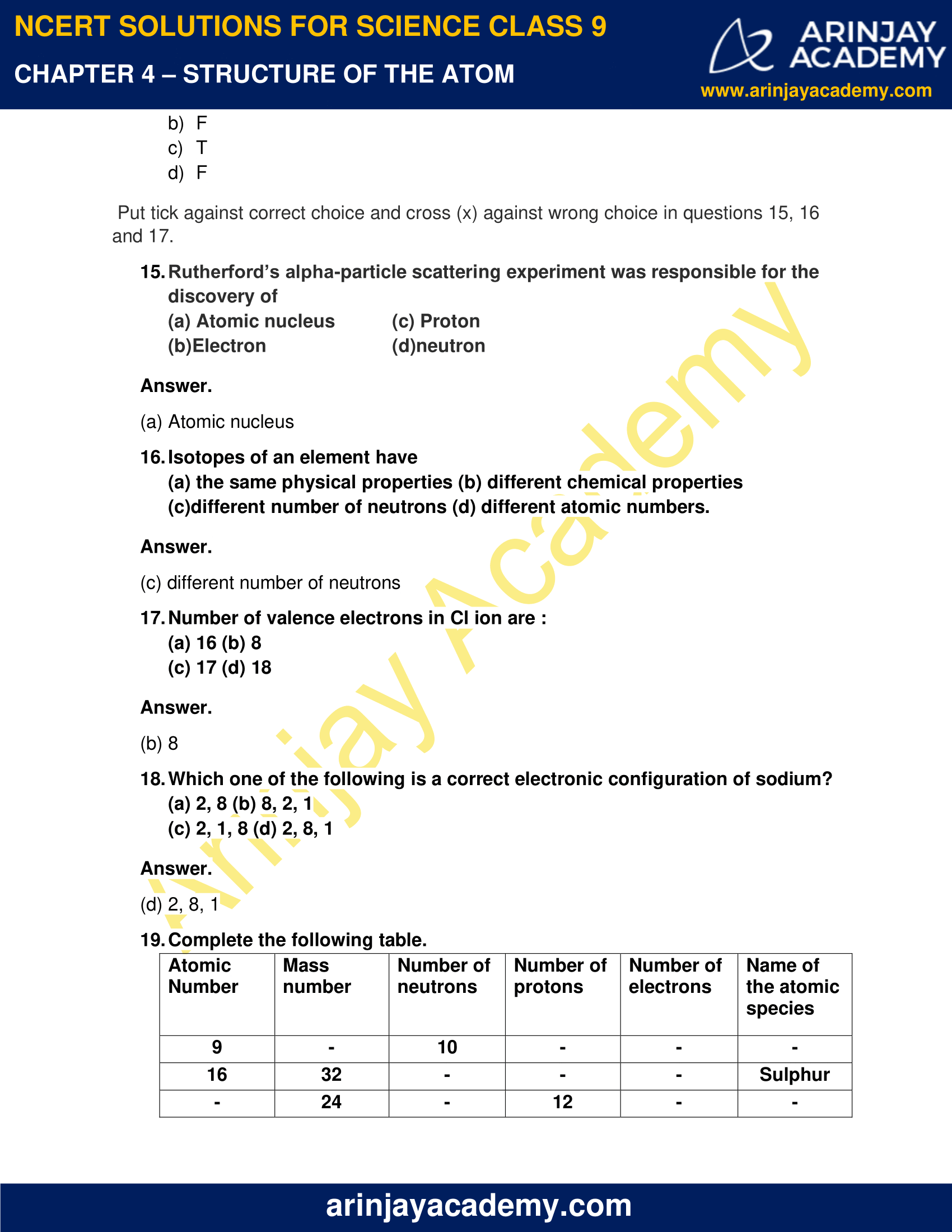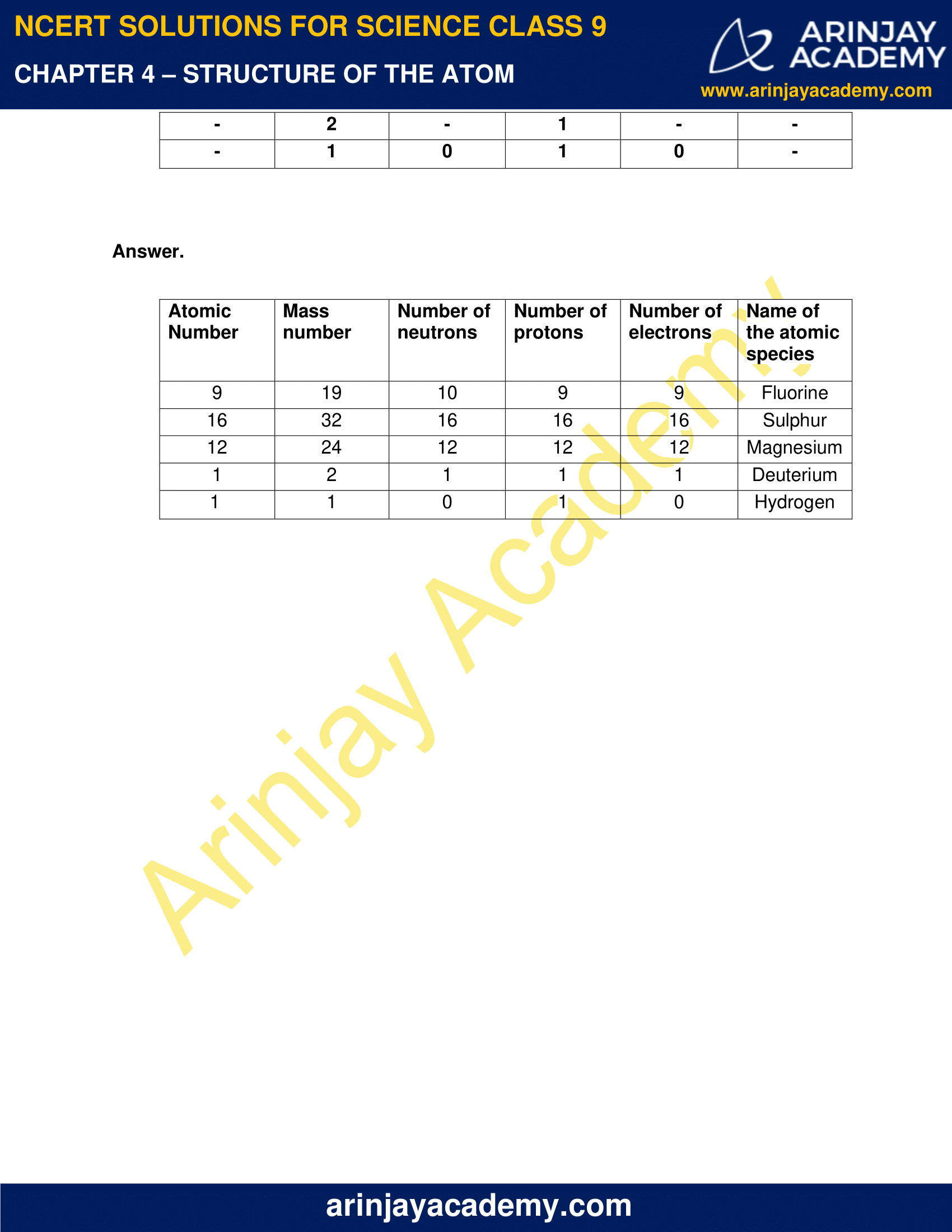### NCERT Solutions for Class 9 Science Chapter 4 – Structure of the Atom – NCERT Exercises

NCERT Solutions for Class 9 Science Chapter 4 – Structure of the Atom, Exercises includes all in text and exercise solved questions which helps you to understand the topic covered in Chapter 4 Structure of the Atom, in a better manner to help you to score good marks in your examinations.

### NCERT Solutions for Class 9 Science Chapter 4 – Intext Questions – Page 47

1. What are canal rays?

Positively charged radiations produced in the discharge tube containing a gas at low pressure when a high potential difference is applied between the electrodes, are called canal rays. They travel towards the oppositely charged cathode.       `

2. If an atom contains one electron and one proton, will it carry any charge or not?

No, the atom will not carry any charge because electron carries one-unit negative charge while the proton carries one unit positive charge. Thus, the net charge of the atom will be zero.

### NCERT Solutions for Class 9 Science Chapter 4 – Intext Questions – Page 49

1. On the basis of Thomson’s model of an atom, explain how the atom is neutral as a whole.

According to Thomson’s model, an atom consists of a positively charged sphere and the electrons are embedded in it like seeds in a watermelon. The total negative charge is equal to the total positive charge. Hence, an atom is neutral as a whole.

2. On the basis of Rutherford’s model of an atom, which sub-atomic particle is present in the nucleus of an atom?

Protons

3. Draw a sketch of Bohr’s model of an atom with three shells.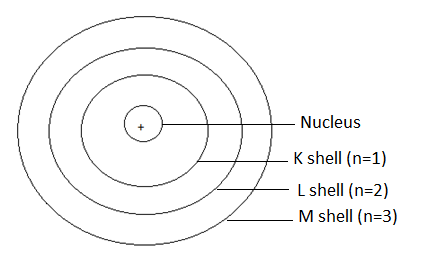4. What do you think would be the observation if the α-particle scattering experiment is carried out using a foil of metal other than gold?

If the α-particle scattering experiment is carried out by using foil of any other metal, there would be no change in the observations. But since other metals are not so malleable, such a thin foil is difficult to obtain. In a thick foil, more α-particles would bounce back and location of positive mass inside the atom would not be detected. So, use of gold foil is preferred.

### NCERT Solutions for Class 9 Science Chapter 4 – Intext Questions – Page 49:

1. Name the three sub-atomic particles of an atom.

The sub-atomic particles of an atom are- Electron, Proton and Neutron.

2. Helium atom has an atomic mass of 4u and two protons in its nucleus. How many neutrons does it have?

Atomic mass of Helium= 4u

Number of protons = 2

Let the number of neutrons = x

Number of protons + Number of neutrons = Atomic mass

2 + x = 4 Thus, x = 2

Helium has 2 neutrons.

### Page 50

1. Write the distribution of electrons in carbon and sodium atoms.

Atomic number of carbon is 6, So number of electrons = 6.

Hence, 1st shell (K-shell) would have 2 electrons and the remaining electrons i.e. 4 will be present in the 2nd shell (L-shell)

K, L (2,4)

Atomic number of sodium is 11, So number of electrons = 11.

Hence, 1st shell (K-shell) would have 2 electrons, 8 electrons will be present in the 2nd shell (L-shell) and the remaining last electron will be present in 3rd shell(M-shell)

K, L, M (2,8,1)

2. If K and L shells of an atom are full, then what would be the total number of electrons in the atom?

Maximum number of electrons in K-shell = 2

Maximum number of electrons in L-shell = 8

So, when both K and L shells are full, total number of electrons present in the atom = 2+8 = 10.

### Page 52

1. How will you find the valency of chlorine, sulphur and magnesium?

Atomic number of Chlorine = 17

Distribution of electrons = K, L, M (2, 8, 7)

The outermost shell has 7 electrons and can easily complete its octet by gaining 1 electron. Hence, the valency = – 1.

Atomic number of Sulphur = 16

Distribution of electrons = K, L, M (2, 8, 6)

The outermost shell has 6 electrons and can easily complete its octet by gaining 2 electrons. Hence, the valency= – 2.

Atomic number of Magnesium= 12

Distribution of electrons = K, L, M (2, 8, 2)

The outermost shell has 2 electrons and can easily lose these two electrons to complete its octet. Hence, the valency= + 2.

2. If the number of electrons in an atom is 8, and the number of protons is also 8, then

i. What is the atomic number of the atom?
ii. What is the charge of the atom?

i. Atomic number of atom = Number of protons = 8
ii. As the number of protons is equal to the number of electrons, so the net charge on the atom will be zero.

3. With the help of table 4.1, find out the mass number of oxygen and sulphur atom.

Mass number = mass of protons + mass of electrons

Mass number of oxygen= 8 + 8 = 16

And mass number of sulphur = 16 + 16= 32

### NCERT Solutions for Class 9 Science Chapter 4 – Intext Questions – Page 53

1. For the symbol H, D and T tabulate three sub-atomic particles found in each of them.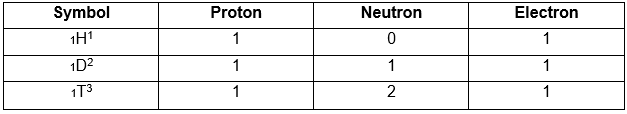2. Write the electronic configuration of any one pair of isotopes and isobars.

Isotopes of Chlorine (atoms with same number of protons but the number of neutrons differ) are 17Cl35 and 17Cl37

Electronic configuration of each of them = K, L, M (2, 8, 7)

Isobars have same mass but different atomic numbers. For example: 18Ar40 and 20Ca40.

Electronic configuration of 18Ar40 = K, L, M (2, 8, 8)

Electronic configuration of  20Ca40 = K, L, M, N (2, 8, 8,2)

### NCERT Solutions for Class 9 Science Chapter 4 – NCERT Exercises – Page 54

1. Compare the properties of electrons, protons and neutrons.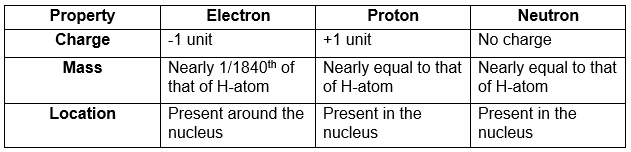2. What are the limitations of J.J. Thomson’s model of the atom?

Limitations of J.J Thomson’s model are-

i. J.J. Thomson’s model could not explain the results of the scattering experiments carried out by Rutherford.
ii. It could not explain the stability of an atom.

3. What are the limitations of Rutherford’s model of the atom?

Limitations of Rutherford’s model of an atom are-

i. Rutherford’s model could not explain why a moving charge (electron) does not lose energy and fall into the nucleus.
ii. It could not explain how the electrons are distributed in the atom.

4. Describe Bohr’s Model of the atom.

The main postulates of Bohr’s model of an atom are-

i. Electrons revolve around the nucleus in orbits called energy levels
ii. The orbits are represented by letters K, L , M etc or numbers 1, 2,3 and so on.
iii. While revolving around the nucleus, electrons do not radiate energy.
iv. Energy is lost or gained by an electron only when it jumps from one orbit to the other.

5. Compare all the proposed models of an atom given in the chapter.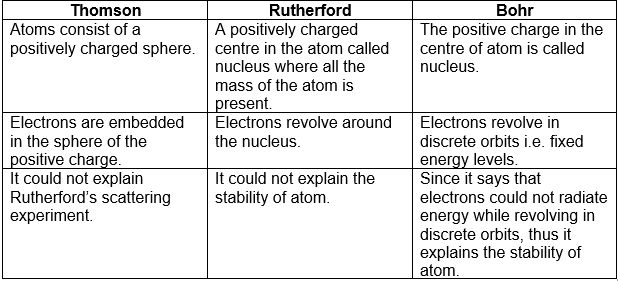6. Summarize the rules for writing of distribution of electrons in various shells for the first eighteen elements.

Rules for writing of distribution of electrons in various shells are-

i. The maximum number of electrons that can be present in nth shell = 2n2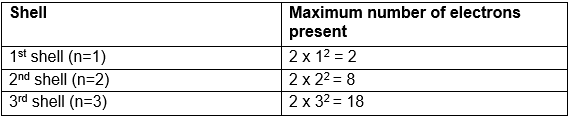ii. The outermost shell cannot have more than 8 electrons.

iii. Electrons do not enter a new shell unless the inner shells are completely filled.

7. Define valency by taking examples of silicon and oxygen.

Valency is defined as the number of electrons which an atom can loose or gain or share with other atoms to complete its octet.

Examples-

Oxygen Atomic number = 8

Number of electrons present = 8

Electronic configuration K, L (2, 6)

The outermost shell contains 6 electrons and will accept 2 electrons to complete its octet. Thus valency = 2

Silicon Atomic number = 14

Number of electrons present = 14

Electronic configuration K, L, M (2, 8, 4)

The outermost shell contains 4 electrons and can share it with other atoms to complete its octet. Thus valency = 4

8. Explain with examples

i. Atomic number
ii. Mass Number
iii. Isotopes and
iv. Isobars. Give any two uses of isotopes.

i. Atomic number: The number of protons present in the nucleus of an atom is called its atomic number. For example. Sodium atom has 11 protons thus its atomic number is 11

ii. Mass Number: The total number of protons and neutrons present in the nucleus of an atom is called its mass number. For example Sodium atom has 11 protons and 12 neutrons, So the mass number = 11 + 12 = 23

iii. Isotopes: Atoms of an element having same atomic number but different mass number are called isotopes. For example Hydrogen atom has three isotopes- 1H1, 1H2, 1H3

iv. Isobars: Atoms of different elements with different atomic numbers but same mass number are called isobars. For example 18Ar40 and 20Ca40 are isobars

Uses of isotopes are –

1. An isotope of Uranium (U-235) is used as a fuel for the production of electricity.
2. An isotope of cobalt is used in the treatment of cancer.

9. Na+ has completely filled K and L shells. Explain.

Atomic number of Na = 11 i.e. it has 11 electrons.

Electronic configuration will be as follows-

1st shell K (n=1) can have maximum electrons = 2n2 = 2(1)2 = 2

2nd shell L (n=2) can have maximum electrons = 2n2 = 2(2)2 =8

The remaining one electron will enter into the 3rd shell. Thus K and L shells are completely filled even if one electron is lost to form Na+.

10. If bromine atom is available in the form of, say, two isotopes 7935Br (49.7%) and 8135Br (50.3%), calculate the average atomic mass of bromine atom.

% of bromine isotope with atomic mass 79 = 49.7 %

% of bromine isotope with atomic mass 81 = 50.3 %

Average atomic mass of bromine atom = (79x 49.7 + 81x 50.3) /100 = 80.006u

11. The average atomic mass of a sample of an element X is 16.2 u. What are the percentages of isotopes 168X and 188X in the sample?

Let the percentage of isotope 168X = z

Then, the percentage of isotope 188X = 100- z

Average atomic mass = 16 x z + 18(100-z) / 100 = 18 – 0.02x

=16.2 = 18 – 0.02 (Given)

So, z= 90% i.e. Percentage of isotope 168X = 90%

And, percentage of isotope 188X = 100 – 90 = 10%

12. If Z = 3, what would be the valency of the element? Also, name the element.

Element with Z=3 is Lithium (Li)

Electronic Configuration = K, L (2, 1)

The outermost shell has one electron, so the valency is 1.

13. Composition of the nuclei of two atomic species X and Y are given as under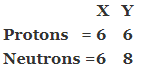Give the mass number of X and Y. What is the relation between the two species?

Mass number = Mass of protons + Mass of neutrons

Mass of  X = 6 + 6 = 12

Mass of  Y = 6 + 8= 14

As both contain the same number of protons (i.e. 6) therefore, their atomic number is same (i.e. 6), but they have different mass numbers. Hence, they are isotopes.

14. For the following statements, write T for True and F for False.
(a) J.J. Thomson proposed that the nucleus of an atom contains only nucleons.
(b) A neutron is formed by an electron and a proton combining together. Therefore, it is neutral.
(c) The mass of an electron is about 1/2000 times that of proton.
(d) An isotope of iodine is used for making tincture iodine, which is used as a medicine.

a) F
b) F
c) T
d) F

Put tick against correct choice and cross (x) against wrong choice in questions 15, 16    and 17.

15. Rutherford’s alpha-particle scattering experiment was responsible for the discovery of
(a) Atomic nucleus
(b)Electron
(c) Proton
(d)neutron

(a) Atomic nucleus

16. Isotopes of an element have
(a) the same physical properties
(b) different chemical properties

(c)different number of neutrons
(d) different atomic numbers.

(c) different number of neutrons

17. Number of valence electrons in Cl ion are :
(a) 16
(b) 8

(c) 17
(d) 18

(b) 8

18. Which one of the following is a correct electronic configuration of sodium?
(a) 2, 8
(b) 8, 2, 1

(c) 2, 1, 8
(d) 2, 8, 1

(d) 2, 8, 1

19. Complete the following table.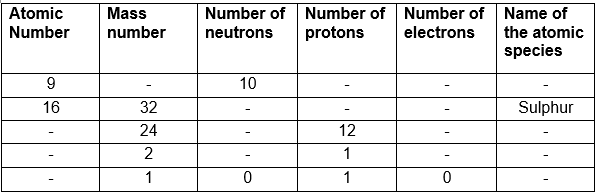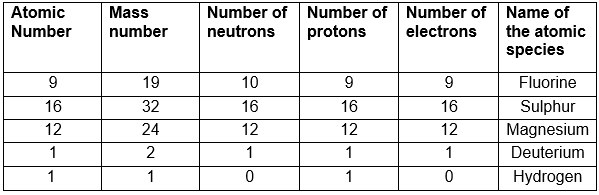### Topics Covered in Structure of the Atom Class 9 Science

1. Charged Particles in Matter
2. The Structure of an Atom
• THOMSON’S MODEL OF AN ATOM
• RUTHERFORD’S MODEL OF AN ATOM
• BOHR’S MODEL OF ATOM
• NEUTRONS
3. How are Electrons Distributed in Different Orbits (Shells)?
4. Valency
5. Atomic Number and Mass Number
6. Isotopes
• ISOBARS